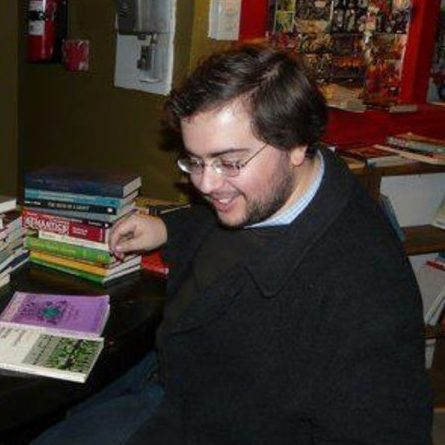Branimir Cacic
(University of New Brunswick)
October 20, 2021
14:00
(CEST)
Organized by Europe GNCG Seminar.

## Classical gauge theory on quantum principal bundles

In his very first note on NC differential geometry, Connes introduced constant curvature connections on Heisenberg bimodules over an irrational NC $2$-torus $\mathcal{A}_\theta$. When $\theta$ is a quadratic irrationality, these Heisenberg bimodules include non-trivial NC line bundles—what, then, is the underlying $U(1)$-gauge theory? We use this case study to demonstrate how approaches to non-universal quantum principal bundles introduced by Brzeziński–Majid and Đurđević, respectively, can be fruitfully synthesized to reframe the basic concepts of gauge theory—gauge transformation, gauge potential, and field strength — in terms of reconstruction of calculi on the total space (to second order) from given calculi on the structure quantum group and base, respectively. In particular, we obtain gauge-equivariant moduli spaces of all suitable first- and second-order total differential calculi, respectively, compatible with these choices.